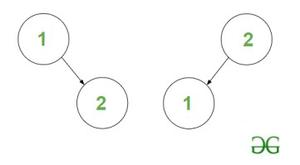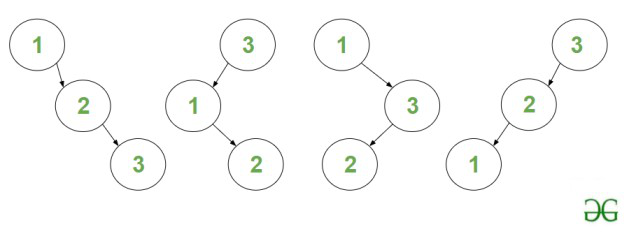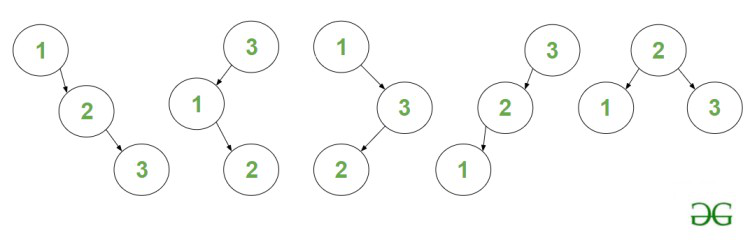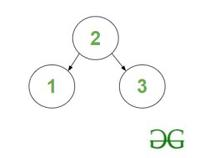# Count of BSTs having N nodes and maximum depth equal to H

Given two integers N and Hthe task is to find the count of distinct Binary Search Trees consisting of N nodes where the maximum depth or height of the tree is equal to H.

Note: The height of BST with only the root node is 0.

Examples:

Input: N = 2, H = 1
Output: 2
Explanation: The two BST’s are :BST’s of height H = 1 and nodes N = 2

Input: N = 3, H = 2
Output: 4
Explanation: The four BST are :BST’s of height H = 2 and nodes N = 3

Naive Approach: The problem can be solved using Recursion which can be memoized to obtain a Dynamic Programming solution based on the following idea:

The problem can be efficiently solved by finding the count of BST’s having maximum depth upto H (ie, [0 – H]) instead of exactly H.

Let f(N, H) represent the count of BST’s consisting of ‘N’ nodes and having maximum depth upto ‘H’. Then the solution for the above problem: count of BST’s having maximum depth of exactly ‘H’ is equal to f(N, H) – f(N, H – 1).

Follow the illustration below for a better understanding.

Illustration:

Consider: N = 3, H = 2

The answer for this example is : count of BST’s of maximum depth upto 2 – count of BST’s of maximum depth upto 1.

• Count of BST’s of maximum depth upto 2 is 5they are:5 – BST’s of maximum depth upto 2

• Count of BST’s of maximum depth upto 1 is 1it is :1 – BST of maximum depth up to 1

• Hence the count of BST’s of maximum depth equal to ‘2’ is 4.

Follow the steps mentioned below to solve the problem.

• The count of BST with Node i as root Node is equal to product of count of BST’s of left subtree formed by nodes 1 to i-1 and right subtree formed by nodes i+1 to N.
• In order to find the count of BST of left subtree, we can recursively call the same function for depth H-1 and N=i – 1. To find the count of BST of right subtree, recursively call the function for depth H-1 and N=Ni.
• Loop over all values ​​of i from [1, N] as root node and add the product of count of left and right subtree to the result.

Time Complexity: O(N * 2N)
Auxiliary Space: O(1)

Efficient Approach: The above approach can be optimized by using Dynamic Programming because the above problem has Overlapping subproblems and an Optimal substructure. The subproblems can be stored in dp[][] table memoization where dp[N][H] stores the count of BST of maximum depth up to H consisting of N nodes. Follow the steps below to solve the problem:

• Initialize a global multidimensional array dp with all values ​​as -1 that stores the result of each recursive call.
• Define a recursive function, say countOfBST(N, H) and perform the following steps.
• Case 1: If N = 0return 1.
• Case 2: If H = 0return true if N = 1.
• If the result of the state dp[N][H] is already computed, return this value dp[N][H].
• Iterate over the range [1, N] using the variable ‘i‘ as root and perform the following operations.
• Multiply the value of recursive functions countOfBST(i – 1, H – 1) and countOfBST(N – i, H – 1). The two functions calculate the count of BST for the left and the right subtree respectively.
• Add the term to the final answer which stores the total count of BSTs possible for all roots from [1, N].
• Print the value returned by the function countOfBST(N, H).

Below is the implementation of the above approach :

## C++

 ` ` `#include ` `using` `namespace` `std;` ` ` `int` `dp;` ` ` `const` `int` `mod = 1000000007;` ` ` `int` `countOfBST(``int` `N, ``int` `H)` `{` ` ` `    ` `    ` `    ``if` `(N == 0) {` `        ``return` `1;` `    ``}` ` ` `    ` `    ` `    ``if` `(H == 0) {` `        ``return` `N == 1;` `    ``}` ` ` `    ` `    ` `    ``if` `(dp[N][H] != -1) {` `        ``return` `dp[N][H];` `    ``}` ` ` `    ` `    ``int` `ans = 0;` ` ` `    ` `    ` `    ``for` `(``int` `i = 1; i <= N; ++i) {` ` ` `        ` `        ` `        ` `        ` `        ``ans += (countOfBST(i - 1, H - 1) * 1LL` `                ``* countOfBST(N - i, H - 1))` `               ``% mod;` ` ` `        ` `        ``ans %= mod;` `    ``}` ` ` `    ` `    ``return` `dp[N][H] = ans;` `}` ` ` `int` `UtilCountOfBST(``int` `N, ``int` `H)` `{` ` ` `    ` `    ``memset``(dp, -1, ``sizeof` `dp);` ` ` `    ` `    ` `    ``if` `(H == 0) {` `        ``return` `(N == 1);` `    ``}` ` ` `    ` `    ``return` `(countOfBST(N, H)` `            ``- countOfBST(N, H - 1)` `            ``+ mod)` `           ``% mod;` `}` ` ` `int` `main()` `{` `    ` `    ``int` `N = 3;` ` ` `    ` `    ``int` `H = 2;` ` ` `    ``cout << UtilCountOfBST(N, H) << endl;` `    ``return` `0;` `}`

Time Complexity: O(N2 * H)
Auxiliary Space: O(N * H)